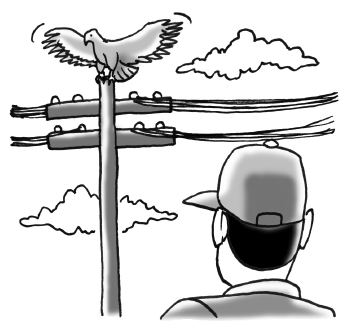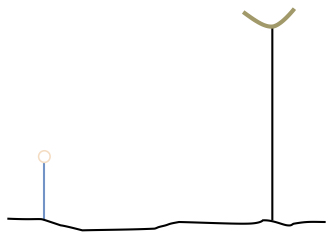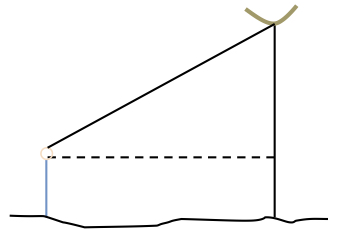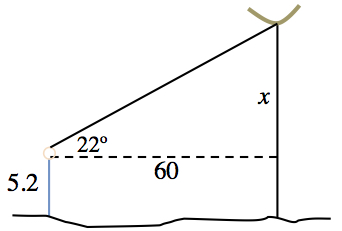### Home > GC > Chapter 4 > Lesson 4.1.5 > Problem4-46

4-46.Leon is standing $60$ feet from a telephone pole. As he looks up, a red-tailed hawk lands on the top of the pole. Leon’s angle of sight up to the bird is $22^\circ$ and his eyes are $5.2$ feet above the ground.

1. Draw a detailed picture of this situation. Label it with all of the given information.

Draw Leon, the telephone pole, and the hawk.

Draw sight and distance lines.

Label the known distance(s) and angle(s). Label the unknown height of the telephone pole $x$.2. How tall is the pole? Show all of your work.

Use the tangent ratio to write an equation and solve for $x$.

$\text{tan}(22^\circ)=\left(\frac{x}{60}\right)$

$60·\tan(22^\circ)=x$
$24.24\approx x$
$\text{height of pole}\approx24.24+5.2\approx29.44\ \text{pies}$